# Excel logical operators: equal to, not equal to, greater than, less than

Many tasks you perform in Excel involve comparing data in different cells. For this, Microsoft Excel provides six logical operators, which are also called comparison operators. This tutorial aims to help you understand the insight of Excel logical operators and write the most efficient formulas for your data analysis.

## Excel logical operators - overview

A logical operator is used in Excel to compare two values. Logical operators are sometimes called Boolean operators because the result of the comparison in any given case can only be either TRUE or FALSE.

Six logical operators are available in Excel. The following table explains what each of them does and illustrates the theory with formula examples.

 Condition Operator Formula Example Description Equal to = =A1=B1 The formula returns TRUE if a value in cell A1 is equal to the values in cell B1; FALSE otherwise. Not equal to <> =A1<>B1 The formula returns TRUE if a value in cell A1 is not equal to the value in cell B1; FALSE otherwise. Greater than > =A1>B1 The formula returns TRUE if a value in cell A1 is greater than a value in cell B1; otherwise it returns FALSE. Less than < =A1= =A1>=B1 The formula returns TRUE if a value in cell A1 is greater than or equal to the values in cell B1; FALSE otherwise. Less than or equal to <= =A1<=B1 The formula returns TRUE if a value in cell A1 is less than or equal to the values in cell B1; FALSE otherwise.

The screenshot below demonstrates the results returned by Equal to, Not equal to, Greater than and Less than logical operators: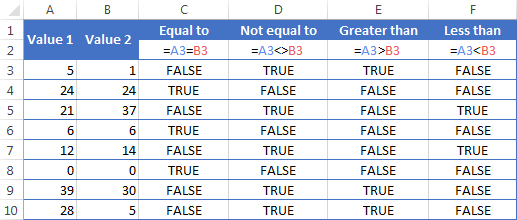It may seem that the above table covers it all and there's nothing more to talk about. But in fact, each logical operator has its own specificities and knowing them can help you harness the real power of Excel formulas.

### Using "Equal to" logical operator in Excel

The Equal to logical operator (=) can be used to compare all data types - numbers, dates, text values, Booleans, as well as the results returned by other Excel formulas. For example:

 =A1=B1 Returns TRUE if the values in cells A1 and B1 are the same, FALSE otherwise. =A1="oranges" Returns TRUE if cells A1 contain the word "oranges", FALSE otherwise. =A1=TRUE Returns TRUE if cells A1 contain the Boolean value TRUE, otherwise it returns FALSE. =A1=(B1/2) Returns TRUE if a number in cell A1 is equal to the quotient of the division of B1 by 2, FALSE otherwise.

#### Example 1. Using the "Equal to" operator with dates

You might be surprised to know that the Equal to logical operator cannot compare dates as easily as numbers. For example, if the cells A1 and A2 contain the date "12/1/2014", the formula `=A1=A2` will return TRUE exactly as it should.

However, if you try either `=A1=12/1/2014` or `=A1="12/1/2014"` you will get FALSE as the result. A bit unexpected, eh?

The point is that Excel stores dates as numbers beginning with 1-Jan-1900, which is stored as 1. The date 12/1/2014 is stored as 41974. In the above formulas, Microsoft Excel interprets "12/1/2014" as a usual text string, and since "12/1/2014" is not equal to 41974, it returns FALSE.

To get the correct result, you must always wrap a date in the DATEVALUE function, like this `=A1=DATEVALUE("12/1/2014")`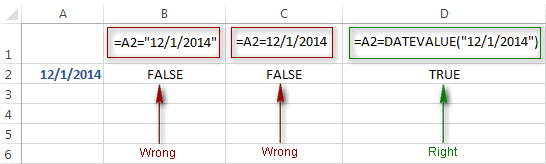Note. The DATEVALUE function needs to be used with other logical operator as well, as demonstrated in the examples that follow.

The same approach should be applied when you use Excel's equal to operator in the logical test of the IF function. You can find more info as well as a few formula examples in this tutorial: Using Excel IF function with dates.

#### Example 2. Using the "Equal to" operator with text values

Using Excel's Equal to operator with text values does not require any extra twists. The only thing you should keep in mind is that the Equal to logical operator in Excel is case-insensitive, meaning that case differences are ignored when comparing text values.

For example, if cell A1 contains the word "oranges" and cell B1 contains "Oranges", the formula `=A1=B1` will return TRUE.

If you want to compare text values taking in to account their case differences, you should use the EXACT function instead of the Equal to operator. The syntax of the EXACT function is as simple as:

EXACT(text1, text2)

Where text 1 and text2 are the values you want to compare. If the values are exactly the same, including case, Excel returns TRUE; otherwise, it returns FALSE. You can also use the EXACT function in IF formulas when you need a case-sensitive comparison of text values, as shown in the below screenshot: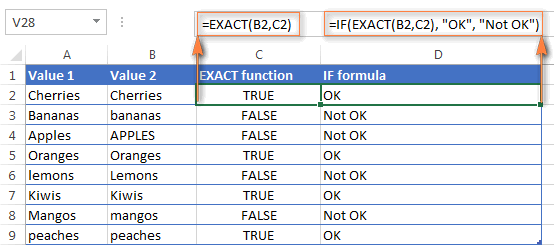Note. If you want to compare the length of two text values, you can use the LEN function instead, for example `=LEN(A2)=LEN(B2)` or `=LEN(A2)>=LEN(B2)`.

#### Example 3. Comparing Boolean values and numbers

There is a widespread opinion that in Microsoft Excel the Boolean value of TRUE always equates to 1 and FALSE to 0. However, this is only partially true, and the key word here is "always" or more precisely "not always" : )

When writing an 'equal to' logical expression that compares a Boolean value and a number, you need to specifically point out for Excel that a non-numeric Boolean value should be treated as a number. You can do this by adding the double minus sign in front of a Boolean value or a cell reference, e. g. `=A2=--TRUE` or `=A2=--B2`.

The 1st minus sign, which is technically called the unary operator, coerces TRUE/FALSE to -1/0, respectively, and the second unary negates the values turning them into +1 and 0. This will probably be easier to understand looking at the following screenshot: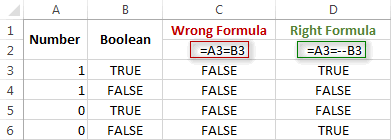Note. You should add the double unary operator before a Boolean when using other logical operators such as not equal to, greater than or less than to correctly compare a numeric and Boolean values.

When using logical operators in complex formulas, you might also need to add the double unary before each logical expression that returns TRUE or FALSE as the result. Here's an example of such a formula: SUMPRODUCT and SUMIFS in Excel.

### Using "Not equal to" logical operator in Excel

You use Excel's Not equal to operator (<>) when you want to make sure that a cell's value is not equal to a specified value. The use of the Not equal to operator is very similar to the use of Equal to that we discussed a moment ago.

The results returned by the Not equal to operator are analogous to the results produced by the Excel NOT function that reverses the value of its argument. The following table provides a few formula examples.

 Not equal to operator NOT function Description =A1<>B1 =NOT(A1=B1) Returns TRUE if the values in cells A1 and B1 are not the same, FALSE otherwise. =A1<>"oranges" =NOT(A1="oranges") Returns TRUE if cell A1 contains any value other than "oranges", FALSE if it contains "oranges" or "ORANGES" or "Oranges", etc. =A1<>TRUE =NOT(A1=TRUE) Returns TRUE if cell A1 contains any value other than TRUE, FALSE otherwise. =A1<>(B1/2) =NOT(A1=B1/2) Returns TRUE if a number in cell A1 is not equal to the quotient of the division of B1 by 2, FALSE otherwise. =A1<>DATEVALUE("12/1/2014") =NOT(A1=DATEVALUE("12/1/2014")) Returns TRUE if A1 contains any value other than the date of 1-Dec-2014, regardless of the date format, FALSE otherwise.

### Greater than, less than, greater than or equal to, less than or equal to

You use these logical operators in Excel to check how one number compares to another. Microsoft Excel provides 4 comparison operates whose names are self-explanatory:

• Greater than (>)
• Greater than or equal to (>=)
• Less than (<)
• Less than or equal to (<=)

Most often, Excel comparison operators are used with numbers, date and time values. For example:

 =A1>20 Returns TRUE if a number in cell A1 is greater than 20, FALSE otherwise. =A1>=(B1/2) Returns TRUE if a number in cell A1 is greater than or equal to the quotient of the division of B1 by 2, FALSE otherwise. =A1

#### Using Excel comparison operators with text values

In theory, you can also use the greater than, greater than or equal to operators as well as their less than counterparts with text values. For example, if cell A1 contains "apples" and B1 contains "bananas", guess what the formula `=A1>B1` will return? Congratulations to those who've staked on FALSE : )

When comparing text values, Microsoft Excel ignores their case and compares the values symbol by symbol, "a" being considered the lowest text value and "z" - the highest text value.

So, when comparing the values of "apples" (A1) and "bananas" (B1), Excel starts with their first letters "a" and "b", respectively, and since "b" is greater than "a", the formula `=A1>B1` returns FALSE.

If the first letters are the same, then the 2nd letters are compared, if they happen to be identical too, then Excel gets to the 3rd, 4th letters and so on. For example, if A1 contained "apples" and B1 contained "agave", the formula `=A1>B1` would return TRUE because "p" is greater than "g".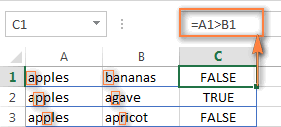At first sight, the use of comparison operators with text values seems to have very little practical sense, but you never know what you might need in the future, so probably this knowledge will prove helpful to someone.

## Common uses of logical operators in Excel

In real work, Excel logical operators are rarely used on their own. Agree, the Boolean values TRUE and FALSE they return, though very true (excuse the pun), are not very meaningful. To get more sensible results, you can use logical operators as part of Excel functions or conditional formatting rules, as demonstrated in the below examples.

#### 1. Using logical operators in arguments of Excel functions

When it comes to logical operators, Excel is very permissive and allows using them in parameters of many functions. One of the most common uses is found in Excel IF function where the comparison operators can help to construct a logical test, and the IF formula will return an appropriate result depending on whether the test evaluates to TRUE or FALSE. For example:

`=IF(A1>=B1, "OK", "Not OK")`

This simple IF formula returns OK if a value in cell A1 is greater than or equal to a value in cell B1, "Not OK" otherwise.

And here's another example:

`=IF(A1<>B1, SUM(A1:C1), "")`

The formula compares the values in cells A1 and B1, and if A1 is not equal to B1, the sum of values in cells A1:C1 is returned, an empty string otherwise.

Excel logical operators are also widely used in special IF functions such as SUMIF, COUNTIF, AVERAGEIF and their plural counterparts that return a result based on a certain condition or multiple conditions.

You can find a wealth of formula examples in the following tutorials:

#### 2. Using Excel logical operators in mathematical calculations

Of course, Excel functions are very powerful, but you don't always have to use them to achieve the desired result. For example, the results returned by the following two formulas are identical:

IF function: `=IF(B2>C2, B2*10, B2*5)`

Formula with logical operators: `=(B2>C2)*(B2*10)+(B2<=C2)*(B2*5)`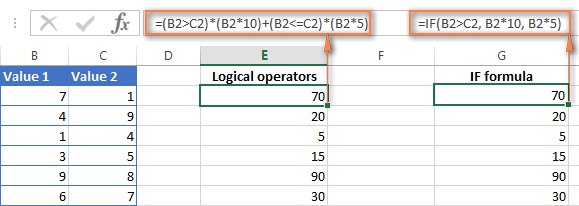I guess the IF formula is easier to interpret, right? It tells Excel to multiply a value in cell B2 by 10 if B2 is greater than C2, otherwise the value in B1 is multiplied by 5.

Now, let's analyze what the 2nd formula with the greater than and less than or equal to logical operators does. It helps to know that in mathematical calculations Excel does equate the Boolean value TRUE to 1, and FALSE to 0. Keeping this in mind, let's see what each of the logical expressions actually returns.

If a value in cell B2 is greater than a value in C2, then the expression B2>C2 is TRUE, and consequently equal to 1. On the other hand, B2<=C2 is FALSE and equal to 0. So, given that B2>C2, our formula undergoes the following transformation: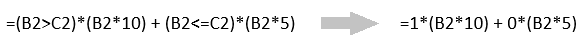Since any number multiplied by zero gives zero, we can cast away the second part of the formula after the plus sign. And because any number multiplied by 1 is that number, our complex formula turns into a simple =B2*10 that returns the product of multiplying B2 by 10, which is exactly what the above IF formula does : )

Obviously, if a value in cell B2 is less than in C2, then the expression B2>C2 evaluates to FALSE (0) and B2<=C2 to TRUE (1), meaning that the reverse of the described above will occur.

#### 3. Logical operators in Excel conditional formatting

Another common use of logical operators is found in Excel Conditional Formatting that lets you quickly highlight the most important information in a spreadsheet.

For example, the following simple rules highlight selected cells or entire rows in your worksheet depending on a value in column A:

Less than (orange): `=A1<5`

Greater than (green): `=A1>20`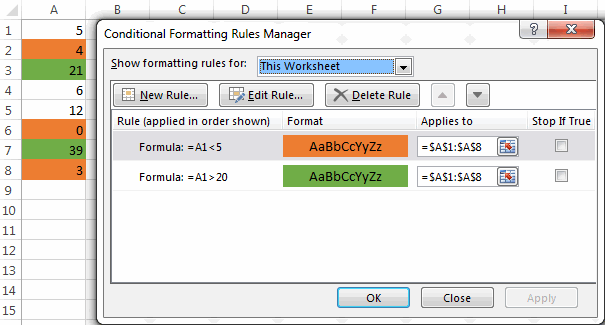For the detailed-step-by-step instructions and rule examples, please see the following articles:

As you see, the use of logical operators in Excel is intuitive and easy. In the next article, we are going to learn the nuts and bolts of Excel logical functions that allow performing more than one comparison in a formula. Please stay tuned and thank you for reading!

## You may also be interested in

### 1,158 comments to "Excel logical operators: equal to, not equal to, greater than, less than"

1.Ahmad says:

Hi..
The formula in column C is IF(A1=B1,1,0)

B B 1
C C 1
B B 1
A A  0
C C 1
A D  0
C B 0
A A  0
D D  0
D D  0
B B 1
A D  0

Why it is returning zero for equal values... like D D should return 1

•Alexander Trifuntov (Ablebits Team) says:

Hi!
Check the values in the cells. Perhaps they contain extra spaces or non-printing characters

2.Waqar says:

What formulae do i use to get the performance status as per below matrix

% Achieved Market Performance Status
100% Top Performer
Above 90% Satisfactory Performer
Below 90% Unsatisfactory Performer

•Alexander Trifuntov (Ablebits Team) says:

Hi!
Explain in detail what result you want to get.

3.Vytautas says:

Hello I have a formula in mind, but I can't write it down in excel maybe you can help me with it.
We have a range between 500 and 600, if a result is lower than 500 then its equal 0, but if its higher or equal than 500 we multiply the result by 2. But maximum result be multiplied by 2 can be up to 600. So if we have a result 700, only 600 will be multiplied by 2.

Do you know what I mean?

4.karrl says:

what formula do I use for table type fee schedule like values greater than 0 but not less than or equal to 500 and get 30 for its answer? please help me

5.Oriya says:

How to compare. ...true r false....example

F1. G1. Diff

Timestamp. TIME_STAMP

6.VAJ says:

Hi! What formula can be used for:

- Let's say the salary is in column G55.
- So, G55 > \$1,600 but < \$1,500...contribution should detected as \$6.20.

•VAJ says:

Thank you! The article was a great help!!

7.Jacob says:

Need one help

Example:

I have INR 400 .

I need following logic

1St INR 200 should be allocated in 35%
1St INR 80 should be allocated in 30%
and balance to be allocated in 35%

8.APK says:

Hi,

I want to achieve the following please:
cell D1 and E1 have different dates and the result of the dates is in cell F1 with Today() function.
cell G1 have a text "Done", whenever type "Done" in G1, it should reflect to cell F1 by ignoring whatever in cell F1.

•Alexander Trifuntov (Ablebits Team) says:

Hi!
From your description it is very difficult to understand what is written in cell F1. But you can completely replace the contents of this cell, ignoring everything in it, only with the help of a VBA macro.
We have already written many times that an Excel formula can only change the cell in which it is written.

•APK says:

CELL"D" CELL"E" CELL"F" =E1-TODAY() CELL"G"
17/01/2022 25/04/2022 67 Days Done
20/01/2022 09/03/2022 20 Days

above example,
Cell F have the difference of Cell E and Today() in days, so when I type "Done" in Cell G,
Cell F values (67Days) to be replaced as "Done"

•Alexander Trifuntov (Ablebits Team) says:

If I understand your task correctly, the following formula with IF function should work for you:

=IF(G1="Done","Done",E1-TODAY())

•APK says:

Thank you - That's what I want - Excellent

Thanks a million.

9.Mohina Saxena says:

Hello all,

I really need urgent help with regards to if operator. I have some dates in column A and other in column B. I want to keep the maximum of both the dates. So I use the following code: IF (A1>B1, A1,B1). While this works beautifully when we dont have blanks or hyphens. But in some case where a cell is having a hyphen it gives me a hyphen again in my new generated column, which I dont want, I want whatever available year is there in either column A or column B it should come out in my new generated column.

A B C [if A>=B, A,B)
1990 1995 1995
2000 - - [I want this to be as 2000]

What is problematic when I go for a <= code in the same shown example then I do get whatever the available year is. By this I mean the following:

A B C [if A<=B, A,B)
1990 1995 1990
2000 - 2000[I want this to be as 2000]

Why this discrepancy is happening, where am I going wrong here? Is something there that I am missing out?

P.S. Since my analysis has some other connected codes in the same column, I cannot delete hyphens and keep them as blanks. And neither I can use a max code because max gives you 0 in case both the columns A and B have blanks which again is not desirable for me.

•Alexander Trifuntov (Ablebits Team) says:

Hello!
You are comparing a number with a character and therefore get the wrong result. Use the ISNUMBER function to test a value for a number.

=IF(IF(ISNUMBER(A1),A1,0)>IF(ISNUMBER(B1),B1,0),A1,B1)

10.Andrew says:

Hi Alexander,

In the first tab I have a list of words "orange" Cell A1 , "banana" CellA2, "pear" cell A3 etc. In the second tab I have a number of other words "ba" CellA1, "ae" CellA2, "xg" Cell A3 etc. I need to write a formula to compare each word in the first tab against all the words in the second tab and if there is a match to any of the words, to return True/False,

For example,
A1 Orange = False (not matches to anything in the second tab)
A2 Banana = TRUE (matches to 'ba' in cell A1 - second tab)
A3 Pear = TRUE (matches to 'ea' in the Cell A2 - second tab)

What formula do you think I can use to do that?

Thank you!

•Alexander Trifuntov (Ablebits Team) says:

Hello!
If I understand your task correctly, the following formula should work for you:

=IF(SUM(IFERROR(SEARCH(Sheet1!A1,Sheet2!\$A\$1:\$A\$10)*(Sheet2!\$A\$1:\$A\$10<>""),0)) + SUM(IFERROR(SEARCH(Sheet2!\$A\$1:\$A\$10,Sheet1!A1)*(Sheet2!\$A\$1:\$A\$10<>""),0)) > 0,TRUE,FALSE)

•Andrew says:

Thank you! This is amazing, it worked!

11.Liyanage De Silva says:

Assume that there are 3 independent columns in excel with both plus and negative values. The first two columns need to be added and the sign (positive or negative) of the result is noted. Also, note the sign of the 3rd column value on the same row. Then both signs should be compared. If both signs are the same then print “c” on the 4th column. If the signs are different print “w” on the 4th column. Take the 3rd column zero value as a negative value in case you get a zero on the 3rd column. Thanks for your help.

•Alexander Trifuntov (Ablebits Team) says:

Hi!

=IF((A1+B1)*IF(C1=0,-1,C1) > 0,"C","W")

12.EMIEL says:

IF MY PARAMETERS ARE: IF A SQUAR IS(<than 1M IN WIDTH and < than 25M IN LENTH and <than100M2 in squares( IT WILL BE "patching" otherwise it will be "milling"

What would be the formula

13.krrishna says:

I have 4 values in different cells MP-50, UP-20,HP-40 AND AP-6 . how to compare a cell value is less than or equal to this value . If a cell contain MP-7 than pls give me formula to solve this

•Alexander Trifuntov (Ablebits Team) says:

Hi!
How do you want to compare text values? What result do you want to get exactly?

14.khuram shahzad says:

Description A/C Description A/C
Opening Balance Opening Balance Assets
Rolling Chair Rolling Chair Assets
Color & Brush Color & Brush Assets
Steel Nail Steel Nail Assets

I want to copy A/C as entering description in first column and it auto collect A/C from last column

•Alexander Trifuntov (Ablebits Team) says:

Hi!
I don't really understand what you want to do. Perhaps you should pay attention to the VLOOKUP function.

15.Mohammed abdul mateen says:

Dear sir,
kindly help to set formula in excel for to compare two value which is greater than the grater value will be multiple to other value in between two value

•Alexander Trifuntov (Ablebits Team) says:

Hi!

•mohammed abdul mateen says:

Dear sir,
if Cell A1 and B1 is comparing with these two which is a greater value in between A1 and B1 to multiple with other value or cell example A1B1 whichever is greater the greater cell is multiple with C1

•Alexander Trifuntov (Ablebits Team) says:

Hi!
I asked you to write an example. This means using numbers and writing in which cells they are written. What are you comparing A1 and B1 to? What is A1B1?

•Mohammed abdul mateen says:

Example ( A:1 100 box, B:1 50 box, if i want to take in this which is greater in these two to price for boxes which one is higher box quantity to multiple with a reasonable amount to the supplier suppose
if A:1 have 100 box B:1 have 50 box
greater of these two A:1><B:1 to detect automatically which one is greater and then to multiple as per price

•Alexander Trifuntov (Ablebits Team) says:

Hi!
I’m sorry but your description doesn’t give me a complete understanding of your task. However, I’ll try to guess and offer you the following formula:

=IF(A1>B1,G1*C1,G1*D1)

•Mohammed abdul mateen says:

Thanks a lot, Sir its work and it help me your genius thank you very much sir very very thanks

16.kb says:

my D column has two options: Apple, Orange
my E column is the dispense
My F column is the due date; based on type and dispense date

Question:
If D says apple then my F is 21 days from E
If D says orange then my F is 28 days from E

How do I format my due date?

•Christian says:

I'm making a computation for a crypto game, what is the proper formula for this? I want to leave my battle result column blank but it's still showing "Lose". Can anyone help me with this? Thanks in advance!

=IF(E8>3,"Win",IF(E8<3,"Lose",IF(OR(E8=0,ISBLANK(E8))," ")))

This is the formula that I used.

•Alexander Trifuntov (Ablebits Team) says:

Hello!

=IF(E8>3,"Win",IF(OR(E8=0,ISBLANK(E8))," ","Lose"))

•Christian says:

Hi Alexander! I tried the formula above and it worked! I truly appreciate it!

17.Matt Giorgio says:

Hello, I have a specific need which I have not been able to work out through all my trials and searching. I need the fill color of A5 to be yellow if C5 is 240 greater than B5 but less than 351 more than B5. And also need A5 fill color to be red if C5 is greater than 350 more than B5. Is there a formula for this? Example: A5 is yellow fill when B5=2210 and C5=2505, and A5 is red fill when B5=1965 and C5=2475. Is there a formula out there for this? I appreciate any help to come my way.

•Alexander Trifuntov (Ablebits Team) says:

Hello!
Create the first conditional formatting rule with condition =C5-B5>240 and the second rule with condition =C5-B5>350. Set the priority of the second rule as described in this guide: Apply several conditional formatting rules to one cell.

18.Souvik Ghosh says:

why this is not working in case of custom data validation =OR((F5"Book1"),(F5"Book2"),(F5"Book3"))

19.lata says:

i am not sure how to do it

20.Jenny says:

If the value in A1 is greater than or equal to 250 but not equal to 400,return 250, and if A1 is greater that or equal to 400 but not equal to 800,return 400, and if the value in A1 is greater than or equal to 800 bit not equal to 2000,return 800.

21.Arlene K Romaska says:

Why oh why does this not work:
IF(AND(\$D\$3="Smith Inc.",\$A\$2=2),C4+D4,C4)

•Arlene K Romaska says:

I'm not sure what's happening when i post my comment, but it's removing the "not equal symbols " The formula should read IF(AND(D3 "does not equal Smith Inc.",A2=2),C4+D4,C\$)

•Alexander Trifuntov (Ablebits Team) says:

Hello!
I don’t know your data source, so I can’t help. Please describe your problem in more detail. It’ll help me understand it better and find a solution for you.

22.Phil says:

I am wanting one cell in the sheet to count the number of times each status is chosen; status was created as "text from drop down list; created list with data validation rule.", the listed choices are: "In Progress", "On Track", "Stalled", "Overdue", "Not Started", and "Complete".

On my reporting sheet, I have created a cell labeled as "Project Status". In this cell, I need a formula that can calculate how many times each status from the list is chosen and the average of those instances. Finally, I want it to calculate the averaged information as an over all status for the project.

•Alexander Trifuntov (Ablebits Team) says:

Hello!
Use the COUNTIF function to calculate how many times each status is applied.
Use the AVERAGEIF function to calculate the average of a condition.

•Phil says:

Much appreciated. I will give that a shot.

23.Rhio says:

I have a table and I want to do advanced filtering to find persons with name only beginning with the letter "B" how would I do this?

24.MARY ERESA CATUBIG VENZON says:

Please help with the formula to be used in counting the number of Students with the range of 75 - 79.

That is the data. Thanks, please

83
83
83
83
83
83
83
77
81
82
75
75
89
89
79
75
79
79
81
91
85
77
76
80
90
88
79
90
80

•Alexander Trifuntov (Ablebits Team) says:

Hello!
You can use array formula

=FREQUENCY(A1:A29,{75,79})

Press Ctrl + Shift + Enter so that array function to work.

It will return 3 values:
The first is the count of numbers is less than or equal to 75
Second, the count of numbers is greater than 75 and less than or equal to 79.
Third - more than 79

25.Richard says:

Can you explain how Excel might be able to perform the following function?

A 'true' cell contains the 24 characters A,B,C,D,E,F,G,H,I,J,K,L,1,2,3,4,5,6,7,8,9,10,11,12. A 'false' cell contains duplicated and omitted characters - such as cell A,A,C,D,E,F,G,H,I,J,K,L,1,1,3,4,5,6,7,8,9,10,11,12.

However, if this false cell A,A,C,D,E,F,G,H,I,J,K,L,1,1,3,4,5,6,7,8,9,10,11,12 is cancelled out by another false cell B,B,C,D,E,F,G,H,I,J,K,L,2,2,3,4,5,6,7,8,9,10,11,12, then these two cells are collectively true because every character occurs the same number of times (twice in this example).

The project I'm working on involves large numbers of these false cells produced by a fairly random process. Can Excel identify sets of complementary cells that are collectively true? (these sets could be pairs - as in the above example - triplets, quadruplets, or more. Note that the whole list needn't be collectively true...just that collectively true sets of cells can be located by searches.)

•Alexander Trifuntov (Ablebits Team) says:

Hello!
If I understood the problem correctly, your task requires a very large number of comparisons. Even if there are 5 cells, the number of possible combinations is 120. This is a complex solution that cannot be found with a single formula. If you have a specific question about the operation of a function or formula, I will try to answer it.

26.Brady says:

I am trying to get a formula to work to pull a price from a different page based on a number on my main page. ex. if the number is greater than 0 but less than 5 it is one price but if it greater than 5 and less than 10 it is another. Any suggestions?

27.Ripunjoy Goswami says:

Hi,

I have the following censored datasets reported during microbiological assay -
< 1
< 4
< 10
< 6
< 5
< 4

Is it possible to determine the mean and SD of such numbers? If yes, how? And how can such numbers be further statistically analyzed?

28.Eb says:

Hi.
I am doing the lateness attendance record. We have 2 shift, morning and evening. For example, those who comes above 0706 and 1906 is late.
If I need to put it into formula, how do I do it?
I was thinking to do =if(A2>0705,"L") but how do I combine it with evening shift.

•Alexander Trifuntov (Ablebits Team) says:

Hello!
The information you provided is not enough to understand your case and give you any advice, sorry.In what format is your time recorded? In text format like number or time format? Give an example of the source data and the expected result.
It’ll help me understand it better and find a solution for you.

•Jenny says:

If the value in A1 is greater than or equal to 250 but not equal to 400,return 250, and if A1 is greater that or equal to 400 but not equal to 800,return 400, and if the value in A1 is greater than or equal to 800 bit not equal to 2000,return 800

29.krishna Rao says:

Use a formula to define the Students Marks Grade as given below in Column G.
>=80 is "A", >=60 to ==35 to =<59 is "C", =<34 is "Not Eligible".

30.brijesh Kumar says:

plz check where is incorect

=IF(AND(L5>80,L585,L590,L595,L5100),I5*100%,0)))))

•brijesh Kumar says:

=IF(AND(L5>80,L585,L590,L595,L5100),I5*100%,0)))))

31.PJ says:

I have a cell that needs to auto calculate an amount if a 25% is not achieved to make the cell equal to 25. Is there a way for excel to auto calculate that value?

Any help on this would be greatly appreciated.

•Alexander Trifuntov (Ablebits Team) says:

Hello!
Sorry, it's not quite clear what you are trying to achieve. From what date do you calculate 25%? Do you want to calculate the sum or set the value to 25? Please describe your problem in more detail. Include an example of the source data and the result you want to get. It’ll help me understand your request better and find a solution for you.

32.John Lim says:

Hi everyone, need help on this problem.

A1 = Karen (Name of Tenant)
B1 = Total Monthly Rental Payment
C1 = Rental Rate per month - 10,000.00
E1 = Less EWT 5%
F1 = PEZA accredited

i want that result on B1 should be non-VAT 12% exclusive if the tenant is PEZA accredited

pls. help me on my current formula

current formula: =(I5+G5)-H5

33.sofian says:

Hi there,

I have some excel question and below is some examples of the question.

I have a pallet and I would like to arrange my box on the this pallet. My Box stacking is not exceed more than 5 feet.

Pallet size is 1100x110x130 in mm.
Box size is 406x345x258 in mm

Base on size dimension above, manual calculation is as below

X : 1100 / 406 = 2.709 (this means only 2 box I only able to arrange in X direction)
Y : 1100 /345 = 3.188 (this means only 3 box I only able to arrange in Y direction)
Z :[ (5ft x 12 x 25.4) - 130] / 258 = 5.403 (this means only able to arrange the Box in 5 layer, Z direction)

Total Box in one pallet is = 2 x 3 x 5
= 30 boxes / pallet

The question is how can I change the answer 2.709 become 2 using excel equation?

34.Eric says:

Formula to return cell (eg A21 value) if value lies between >X<Y where X&Y are cell values. Please! Thanks

35.GD says:

I have huge data with different Values and have to derive at some classification based on three data sets.

Col A & B has Numbers as Values and Col C has Names as Values

Col A has Values ranging from 0 to 1000
Col B has Values ranging from 0 to 1000000
Col C has Values WHITE, RED, ORANGE, BLUE

Could anyone help me to get this please. Below is an example of what I am looking for.

if A is greater than 1 and equals to 4 and is WHITE or RED term it as Medium
if B is greater than 8999 and equals to 16999 term it as Medium

36.April says:

I have a formula that I am stuck building It is using the below information. Can you please advise how to build the "if then" formula cell C5 for the below? I am stuck on trying to get the in between numbers to work. so far I have gotten = IF(C5<4,5,(C5

5 = 0 to 4
4 = 4.01 to 12
3 = 12.01 to 24
2 = 24.01 to 28
1 = 28<

37.AVI says:

I HAVE THREE SHEET, WITH SAME HEADER BUT VARIOUS VALUE IN IT, HOW TO KNOW WHICH SHEET DATA LESS THEN OUT OF THREE. CAN ANY OPTION OR COMMAND TO PRINT LESS SHEET NAME

SHEET NAME
1. 3338 DATA A 1620
2. 5498 DATA A 1550
3. 5913 DATA A 1820

38.SIVA K says:

1+21=22 i need formula of round off one digit 22 = round on 20
2+34=36 this is round on = 40

•Azim Ahamed says:

you can use Mround function.

39.mdc says:

Hi,

What formula should I use for:
If the value is not equal to "follow" in C4 then get the value in C4.

Thanks!

•Alexander Trifuntov (Ablebits Team) says:

Hello!